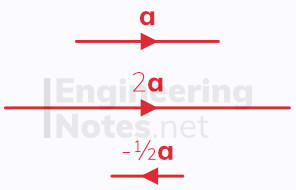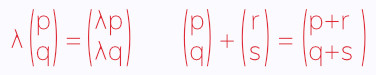#### Notes by Category University Engineering

Electronics*
Mathematics*
Mechanics & Stress Analysis*
Rate these notesNot a fanNot so goodGoodVery goodBrillRate these notes
• A-Level Maths

# Vectors

A vector is a quantity with both magnitude and direction, and is typically represented visually by a line segment between two points.There are many ways of representing vectors in notation:

• The two points that the vector connects with an arrow above them

• As a bold typeface lower case letter

• As an underlined lower case letter

• As a column vector, showing displacement in the x-direction above that in the y-direction

• As a multiple of unit vectors, i (one unit in the positive x-direction) and j (one unit in the positive y-direction)

These notes will predominantly use bold typeface of lower case letters, underlined letters and the unit vectors i and j.Vectors can be multiplied by a scalar, and added and subtracted:## Magnitude & Direction

The magnitude of a vector is given by Pythagoras' Theorem. Magnitude is noted using straight lines on either side of the letter, like modulus.

For the vector a = xi + yj, |a| = √(x²+y²)

A unit direction vector, â, can be found as a / |a|

A vector can also be defined by giving its magnitude and the angle it makes with one of the coordinate axis. This is called magnitude-direction form.